Smartick is an advanced online program that teaches kids math and coding in only 15 min. a day

Aug12

# How to Solve an Addition Problem with Regrouping

Do you know how to solve an addition problem with regrouping? Do you know all the steps and why they’re important? Sometimes you learn how to solve math problems, but you don’t understand why you solve them in a particular way.

This is why we are going to review how to solve an addition problem with regrouping today, and we’ll explain why we solve them the way that we do with visuals.

Let’s do two examples using some math cubes to visualize the problem. We’re going to solve the following addition problems:

## 25 + 18 = ?

First, we’re going to represent the numbers by using math cubes: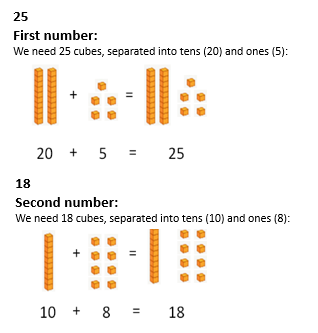Now, we need to put each number into place: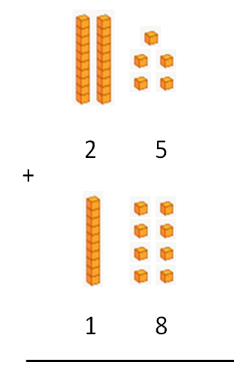First, we will add the ones-place numbers. 5 + 8 gives us 13. 13 is bigger than 10, so we need to separate the number into tens and ones: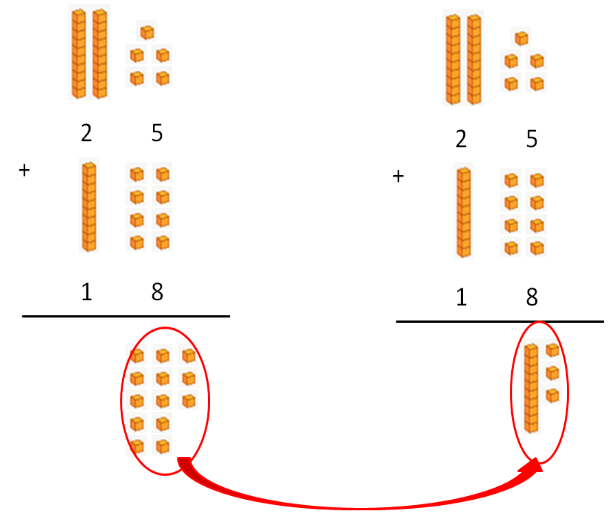Now, we add the tens, remembering that we need to add one ten more; it’s the one that was leftover from when we added the ones-place numbers. This is the “regrouping.”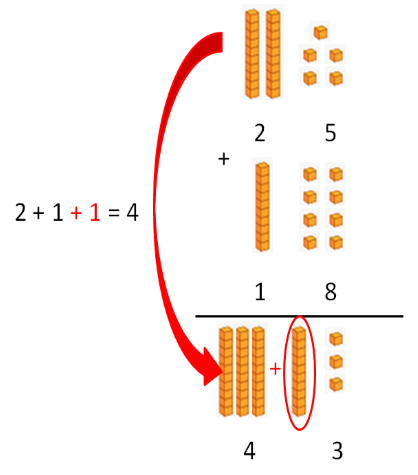Do you get it? Let’s take a look at another example.

## 27 + 4 = ?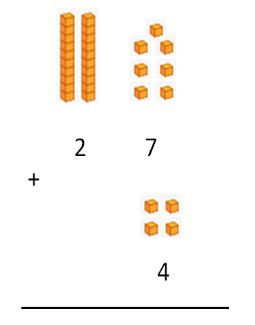7 + 4 is 11, which is greater than 10, so we need to separate the number into tens and ones: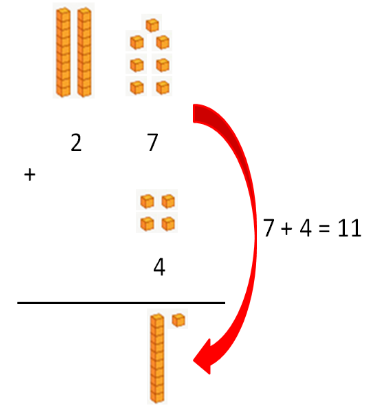Now, we need to add the tens while remembering to add one extra 10.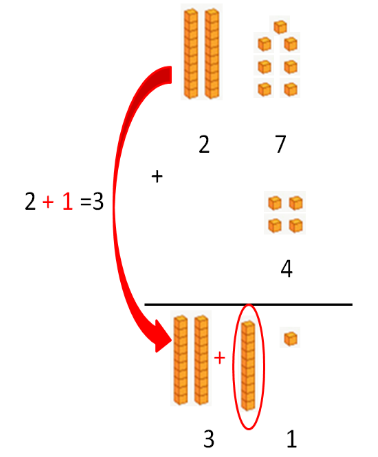Summary: Whenever the ones-place numbers add up to a number greater than 10, we need to carry the tens over to the tens-place column.

If you liked this post, share it with your friends! Remember, at Smartick, you can learn about all kinds of additional problems and much more elementary math!